InfoQ Homepage Articles Getting Started with Machine Learning

Getting Started with Machine Learning

Leia em portuguÃªs

This item in chinese

Data science is fast becoming a critical skill for developers and managers across industries, and it looks like a lot of fun as well. But it’s pretty complicated - there are a lot of engineering and analytical options to navigate, and it’s hard to know if you’re doing it right or where the bear traps lie. In this series we explore ways in to making sense of data science - understanding where it’s needed and where it’s not, and how to make it an asset for you, from people who’ve been there and done it.

This InfoQ article is part of the series "Getting A Handle On Data Science" . You can subscribe to receive notifications via RSS.

Key takeaways

• Most types of Machine Learning projects result in a classification or regression typically using supervised learning approaches.
• Feature engineering is a critical part of most machine learning processes.
• Unsupervised learning algorithms such as K-means can be used to do clustering when you don’t know the data labels beforehand.
• All the majority of the ML algorithms out there do is to find the parameters for their models in a way that best fit the data.
• Overfitting is a common problem data scientists have to face where assumptions from a limited dataset don’t generalise well as more data points were added.

A lot of Machine Learning (ML) projects consist of fitting a (normally very complicated) function to a dataset with the objective of calculating a number like 1 or 0 (is it spam or not?) for classification problems or a set of numbers (e.g., weekly sales of a product) for regression ones. Yes, it's all about numbers and loads of operations which a computer is very good at. That’s the M in ML- what about the L?

Consider the gender recognition by voice dataset which can be found in this Kaggle page. The objective with this dataset is, when given a speech signal, to identify whether it is from a male or female. This challenge falls under the category of a classification problem. The objective here is to assign the class male or female given a speech signal, but classification problems don't necessarily have to be limited to two classes. Some other examples of classification problems are sentiment analysis of text (positive, neutral or negative), image identification (what kind of flower do you see in an image?), etc.

How could the computer learn to identify if a recorded voice is from a male or female? Well, if we want the computer to help us, in this case then we need to speak its language: numbers. In the machine learning world this means extracting features from the data. If you followed the Kaggle link above you can see that they already have extracted lots of features from the speech signal. Some feature examples are: mean frequency, median frequency, standard deviation of frequency, interquartile range, mean of fundamental frequency, etc. In other words, instead of having a time series showing the voice pressure signal, they extracted characteristics of this signal that may help us identify if the voice belongs to a male or female- this is called feature engineering. Feature engineering is a critical part of most machine learning processes.

I will pick two features of this dataset, namely the mean of fundamental frequency and interquartile range, and plot them in the figure below.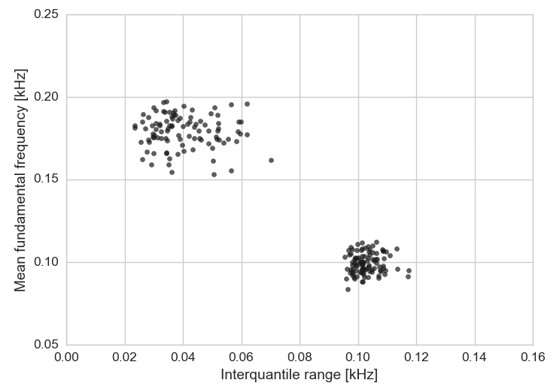Two distinct groups of points appear in this figure. Knowing that this dataset comprises of speech signals from males and females, I guess that the group or cluster of points with higher mean fundamental frequency (higher pitch) belong to females whereas the other cluster belongs to males. Therefore, a possible way to identify which gender the signals belong to is to group the data into two clusters and assign the female label to the cluster with the higher mean fundamental frequency and the male label to the other cluster. It turns out that there are some ML algorithms that do exactly that- clustering. K-means is one of the most used algorithms to perform that operation, where the “K” in the algorithm name is the number of clusters that you want to identify (two in the current case). Notice that all this algorithm takes is an initial number of clusters you want to identify and the raw data and it returns a generic label (0 or 1, for instance), attached to each point, indicating which cluster each instance belongs to. In this case, it was my domain knowledge that assigned meaning to those labels.

K-means belongs to a class of ML algorithms called unsupervised learning where you don’t know your data labels beforehand. It works well here because we have two clearly differentiable clusters but when there is a lot of overlapping might not be the best solution for classification problems. Another class of ML algorithms is called supervised learning, where you make use of the data labels. Let’s take the data labels from the voice recognition dataset and plot again the same figure.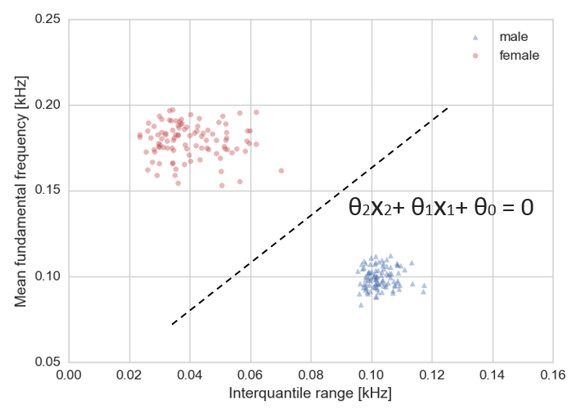The intuition that the cluster with the highest mean fundamental frequency belongs to females was correct after all. To introduce the supervised approach, I'm going to attempt to separate both classes with a line which visually makes sense to me. This line is called decision boundary and I have also written down its equation.  The "thetas" are the line parameters whereas the "x's" correspond to the variables plotted in the graph: interquartile range and mean fundamental frequency, in this case. I’m able to fit this line because I know the data labels. So if I use the left-hand side of the equation on the voice dataset and if the number I get is larger than zero I could predict the voice is from a female, or if this number is below zero, then it's from a male . Easy. Solved.

Not quite.

I plotted only 100 points for each class but the entire dataset is comprised of 3164 data points split half/half between males and females. What happens if I plot 200 data points for each class?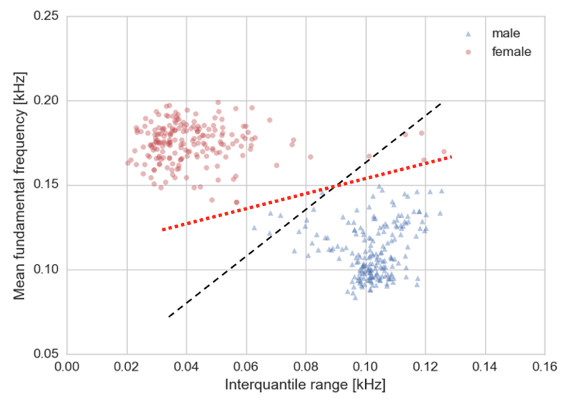Well, the black dashed line now doesn't seem like a good fit. I am going to repeat the process and fit another red dotted line that I visually think could be a good way to separate both classes. The mathematical difference between both lines in the plot is related to the values of the "thetas" from the equation in the first figure. What I did here is to "learn" a better way of separating both classes given the data presented to me. The result, in essence, comes down to finding new coefficients for the decision boundary. You can see where this is going, right?

We are now in a position to understand that the L in ML is related to finding the best parameters to accomplish the objective at hand which, in this case, is to predict if it's a male or female given a voice signal. I'm sorry if I disappointed you, but really what the majority of the ML algorithms out there do is to find the "thetas"/coefficients/parameters for their models in a way that best fit the data (typical examples of such algorithms are Logistic Regression and Artificial Neural Networks). Of course these algorithms do a lot better than my method of visually assigning a line. They are normally based on optimisation functions that fit lines minimising the error between the actual and predicted values by the model. This error is also called loss or loss function in the ML world.

There are also some very widely used algorithms based on collections of decision trees such as Random Forest or Gradient Tree Boosting which don't explicitly find coefficients for a line but find other parameters to split the data resulting in a more complex decision boundary. Actually, the truth is that given the plots shown above, there are no reasons why a line should be chosen as a decision boundary. We could, in theory, fit a curve to the dataset.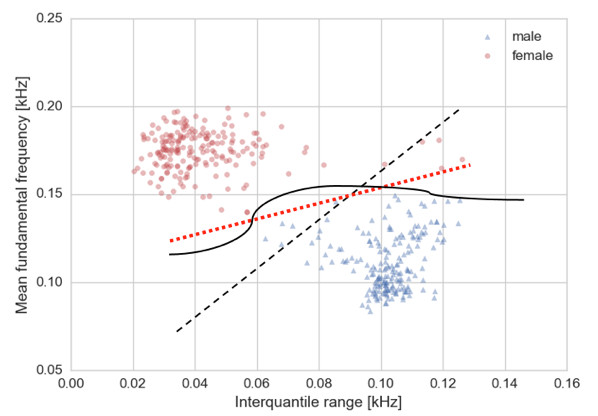The solid black line could be chosen as a decision boundary but there is a problem that haunts pretty much every Data Scientist out there, which is overfitting. What the curve does is to create a decision boundary that is very specific for the current dataset and probably won't generalise well if more data points were added. We have seen the large difference between having 100 or 200 points for each class already. What if even more points are added?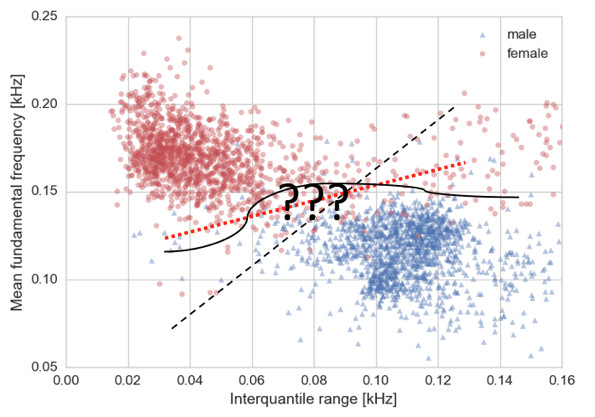It's a mess. The whole dataset is plotted and now my sophisticated method of visually fitting lines to the data becomes very questionable. We need the help of an ML algorithm now to choose a line/ curve which minimizes the error or loss we are committing by fitting these decision boundaries. In addition, we need the help of algorithms here because so far we have shown the dependency of the voice dataset based on two features only, whereas the whole dataset is comprised by 21 features.

This is good and bad news.

Imagine that instead of the two dimensional plot I have shown so far, we had three dimensions where the third dimension is another feature such as the maximum fundamental frequency across the signal (let's call it maxfun). Now imagine the following: every female has maxfun around zero and every male has maxfun around one (this does not happen in this dataset). If we were to plot this in a 3D graph you can easily picture a plane that can split the male and female data in a perfect way. That would have been great. When you add more features to the dataset we can imagine this situation happening with higher dimensional planes (hyper planes) which hopefully will split the data in a more accurate way. This is the good news.

The bad news is that there is no way we can visually check that unless we use dimensionality reduction algorithms such as Principal Component Analysis which transform this highly dimensional data into two or three dimensions, for instance, with the disadvantage that you will no longer keep physical features in the plot axis (like the mean fundamental frequency or maximum fundamental frequency) but have variables that are projections of the most important features of your dataset. Let me illustrate this point by plotting a simple example with the mean frequency and the median frequency of speech from the voice recognition dataset.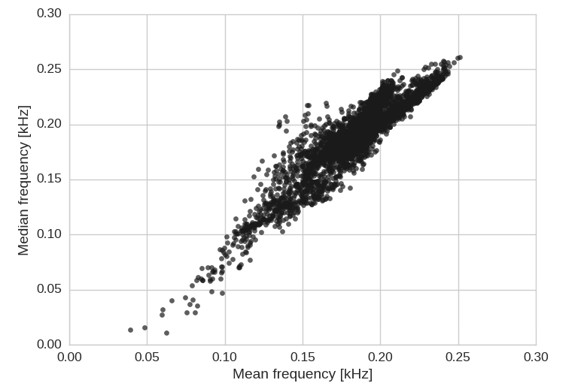The figure shows two correlated variables which makes sense given that they are samples from the same probability distribution of voice frequencies. There is redundant information using these two variables. It would be good to find a way of simplifying the visualisation of the variance of these data in a lower dimension. For that purpose I plot a zoom in version of this figure.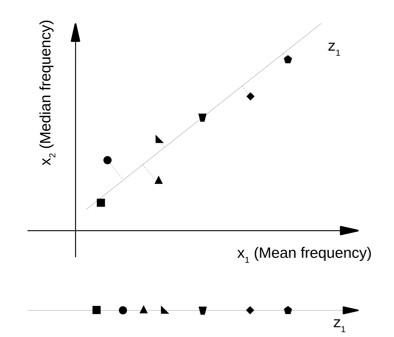What if, instead of visualising the two variables, we visualise the projection of these data into a direction that minimises the loss of information from these two variables? This is done in the z1 axis but still at the expense of some loss of information. For this particular case, this direction can be seen as a frequency, but it’s neither the median or mean. However, imagine applying a projection in a dataset of 21 dimensions into 2. We can’t really know what the meaning of these 2 dimensions is. Moreover, in this simple example not much information was lost but projecting the 21 features into 2 will incur in a substantial loss. It could be interesting in terms of visualization of the data but it shouldn’t be used to perform ML algorithms on.

We have seen that some features in this dataset are correlated; should they be included in the ML project? As a matter of fact, how many features should we use? Using just two features and all the points, the decision boundary does not clearly separate the two classes in this problem. This is confirmed when you calculate the error or loss you incur by using the line as a decision boundary. By adding a third feature, if the feature is adequate, the loss should get lower. If it doesn’t then it is an indication that either this additional feature is not suitable or it’s not important for the problem at hand.

I hope I have awakened your curiosity about ML with this article. This was a general introduction and much more reading is required to develop and understand an ML project from beginning to end. There are ML algorithms written in pretty much every programming language. Python and R are among the most used languages for the job and have very good libraries for ML such as scikit-learn for the former and caret for the latter. I suggest you delve into a tutorial in your language of choice. You know what it’s all about now.Rafael Fernandes is a Data Scientist at Black Swan Data. He has been using Machine Learning to help clients with the pricing and demand of their products as well as to reveal their consumer insights and behaviour. In his previous life, he simulated the re-entry of the Apollo capsule from space at 8 times the speed of sound but he is still an aerospace geek.

Data science is fast becoming a critical skill for developers and managers across industries, and it looks like a lot of fun as well. But it’s pretty complicated - there are a lot of engineering and analytical options to navigate, and it’s hard to know if you’re doing it right or where the bear traps lie. In this series we explore ways in to making sense of data science - understanding where it’s needed and where it’s not, and how to make it an asset for you, from people who’ve been there and done it.

This InfoQ article is part of the series "Getting A Handle On Data Science" . You can subscribe to receive notifications via RSS.

Style

Hello stranger!

You need to Register an InfoQ account or or login to post comments. But there's so much more behind being registered.

Get the most out of the InfoQ experience.

Allowed html: a,b,br,blockquote,i,li,pre,u,ul,p

• Excellent article!

by Andrew Jenkins /

• Great introduction.

by Riaan Perry /

• Excellent article!

Your message is awaiting moderation. Thank you for participating in the discussion.

Very clear explanation

• Great introduction.

by Riaan Perry /

Your message is awaiting moderation. Thank you for participating in the discussion.

Thank you for this good starter article Rafael.

Allowed html: a,b,br,blockquote,i,li,pre,u,ul,p

Allowed html: a,b,br,blockquote,i,li,pre,u,ul,p

Is your profile up-to-date? Please take a moment to review and update.

Note: If updating/changing your email, a validation request will be sent

Company name:
Company role:
Company size:
Country/Zone:
State/Province/Region:
You will be sent an email to validate the new email address. This pop-up will close itself in a few moments.